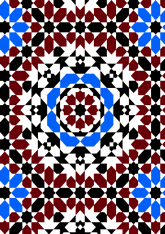data175/MOR2404X

## Geometry

• The symmetry group of the tiling is *442 (p4m).
• All the internal angles of the constituent polygons are a multiple of 45°.
• Contains one square.
• Contains two regular two-pointed star polygons with vertex angle of 45°.
• Contains five regular 8-pointed star polygons with vertex angle of 90°.
• There are 17 non-regular reflective tiles (including one kite and one dart).
• The tiling satisfies the interlace condition and has five finite interlaces and one infinite interlace with straight cross-overs.
• The tiling is edge-to-edge.
• As drawn, contains about 990 polygons.

## References

Publications referenced: